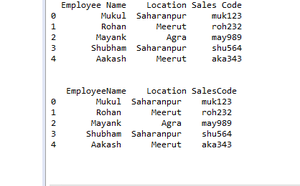Open In App

# Remove spaces from column names in Pandas

Removing spaces from column names in pandas is not very hard we easily remove spaces from column names in pandas using replace() function. We can also replace space with another character. Let’s see the example of both one by one.

Example 1: remove the space from column name

## Python

 `# import pandas``import` `pandas as pd`` ` `# create data frame``Data ``=` `{``'Employee Name'``: [``'Mukul'``, ``'Rohan'``, ``'Mayank'``,``                          ``'Shubham'``, ``'Aakash'``],``         ` `        ``'Location'``: [``'Saharanpur'``, ``'Meerut'``, ``'Agra'``, ``                     ``'Saharanpur'``, ``'Meerut'``],``         ` `        ``'Sales Code'``: [``'muk123'``, ``'roh232'``, ``'may989'``,``                       ``'shu564'``, ``'aka343'``]}`` ` `df ``=` `pd.DataFrame(Data)`` ` `# print original data frame``print``(df)`` ` `# remove special character``df.columns ``=` `df.columns.``str``.replace(``' '``, '')`` ` `# print file after removing special character``print``(``"\n\n"``, df)`

Output:Example 2: replace space with another character

## Python

 `# import pandas``import` `pandas as pd`` ` `# create data frame``Data ``=` `{``'Employee Name'``: [``'Mukul'``, ``'Rohan'``, ``'Mayank'``,``                          ``'Shubham'``, ``'Aakash'``],``         ` `        ``'Location'``: [``'Saharanpur'``, ``'Meerut'``, ``'Agra'``, ``                     ``'Saharanpur'``, ``'Meerut'``],``         ` `        ``'Sales Code'``: [``'muk123'``, ``'roh232'``, ``'may989'``,``                       ``'shu564'``, ``'aka343'``]}`` ` `df ``=` `pd.DataFrame(Data)`` ` `# print original data frame``print``(df)`` ` `# replace space with another character``df.columns ``=` `df.columns.``str``.replace(``' '``, ``'_'``)`` ` `# print file after removing special character``print``(``"\n\n"``, df)`

Output: